# Angle Bisector Theorem

As per the Angle Bisector theorem, the angle bisector of a triangle bisects the opposite side in such a way that the ratio of the two line segments is proportional to the ratio of the other two sides. Thus the relative lengths of the opposite side (divided by angle bisector) are equated to the lengths of the other two sides of the triangle. Angle bisector theorem is applicable to all types of triangles.

Class 10 students can read the concept of angle bisector theorem here along with the proof. Apart from the angle bisector theorem, we will also discuss here the external angle theorem, perpendicular bisector theorem, the converse of angle bisector theorem.

## What is Angle Bisector Theorem?

An angle bisector is a straight line drawn from the vertex of a triangle to its opposite side in such a way, that it divides the angle into two equal or congruent angles.

Angle Bisector Theorems of Triangles

The table below shows the statements related to internal and external angle bisector theorems as well as their converse.

 Theorem Statement Internal angle bisector theorem The angle bisector of a triangle divides the opposite side into two parts proportional to the other two sides of the triangle. Converse of Internal angle bisector theorem In a triangle, if the interior point is equidistant from the two sides of a triangle, then that point lies on the angle bisector of the angle formed by the two line segments. Perpendicular bisector theorem The perpendicular bisector bisects the given line segment into two equal parts, to which it is perpendicular. For a triangle, if a perpendicular bisector is drawn from the vertex to the opposite side, it divides the side into two congruent segments. External angle bisector theorem The external angle bisector divides the opposite side externally in the ratio of the sides containing the angle, and this condition usually occurs in non-equilateral triangles.

Now let us see, what is the angle bisector theorem.

According to the angle bisector theorem, the angle bisector of a triangle divides the opposite side into two parts that are proportional to the other two sides of the triangle.

## Interior Angle Bisector Theorem

In the triangle ABC, the angle bisector intersects side BC at point D. See the figure below.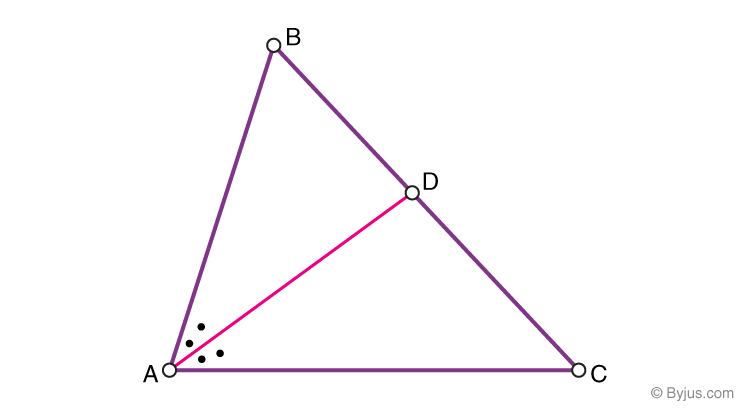As per the Angle bisector theorem, the ratio of the line segment BD to DC equals the ratio of the length of the side AB to AC.

$$\begin{array}{l}\frac{\left | BD \right |}{\left | DC \right |}=\frac{\left | AB \right |}{\left | AC \right |}\end{array}$$

Conversely, when a point D on the side BC divides BC in a ratio similar to the sides AC and AB, then the angle bisector of ∠ A is AD. Hence, according to the theorem, if D lies on the side BC, then,

$$\begin{array}{l}\frac{\left | BD \right |}{\left | DC \right |}=\frac{\left | AB \right |Sin\angle DAB}{\left | AC \right |Sin\angle DAC}\end{array}$$

If D is external to the side BC, directed angles and directed line segments are required to be applied in the calculation.

Angle bisector theorem is applied when side lengths and angle bisectors are known.

### Proof of Angle bisector theorem

We can easily prove the angle bisector theorem, by using trigonometry here. In triangles ABD and ACD (in the above figure) using the law of sines, we can write;

$$\begin{array}{l}\frac{AB}{BD}=\frac{sin\angle BDA}{sin\angle BAD}….(1)\end{array}$$

$$\begin{array}{l}\frac{AC}{DC}=\frac{sin\angle ADC}{sin\angle DAC} ….(2)\end{array}$$

The angles ∠ ADC and ∠ BDA make a linear pair and hence called adjacent supplementary angles.

Since the sine of supplementary angles are equal, therefore,

Sin ∠ BDA = Sin ∠ ADC     …..(3)

Also,

Thus,

Sin ∠ BDA = Sin ∠ ADC    …(4)

Hence, from equations 3 and 4, we can say, the RHS of equations 1 and 2 are equal, therefore, LHS will also be equal.

$$\begin{array}{l}\frac{\left | BD \right |}{\left | DC \right |}=\frac{\left | AB \right |}{\left | AC \right |}\end{array}$$

Hence, the angle bisector theorem is proved.

Condition:

If the angles ∠ DAC and ∠ BAD are not equal, the equation 1 and equation 2 can be written as:

$$\begin{array}{l}\frac{\left | AB \right |}{\left | BD \right |} sin\ \angle BAD = sin\ \angle BDA\end{array}$$
$$\begin{array}{l}\frac{\left | AC \right |}{\left | DC \right |} sin\ \angle DAC = sin\ \angle ADC\end{array}$$

Angles ∠ ADC and ∠ BDA are supplementary, hence the RHS of the equations are still equal. Hence, we get

$$\begin{array}{l}\frac{\left | AB \right |}{\left | BD \right |} sin\ \angle BAD = \frac{\left | AC \right |}{\left | DC \right |} sin\ \angle DAC\end{array}$$

This rearranges to generalized view of the theorem.

## Converse of Angle Bisector Theorem

In a triangle, if the interior point is equidistant from the two sides of a triangle then that point lies on the angle bisector of the angle formed by the two line segments.

## Triangle Angle Bisector Theorem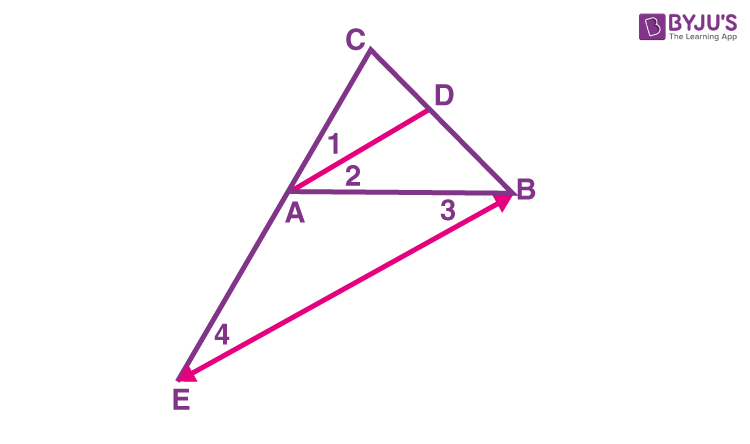Extend the side CA to meet BE to meet at point E, such that BE//AD.

Now we can write,

CD/DB = CA/AE  (since AD//BE)  —-(1)

∠4  =  ∠1          [corresponding angles]

∠1 = ∠2   [AD bisects angle CAB]

∠2 = ∠3    [Alternate interior angles]

∠3 = ∠4  [By transitive property]

ΔABE is an isosceles triangle with AE=AB

Now if we replace AE by AB in equation 1, we get;

CD/DB = CA/AB

Hence proved.

## Perpendicular Bisector Theorem

According to this theorem, if a point is equidistant from the endpoints of a line segment in a triangle, then it is on the perpendicular bisector of the line segment.

Alternatively, we can say, the perpendicular bisector bisects the given line segment into two equal parts, to which it is perpendicular. In the case of a triangle, if a perpendicular bisector is drawn from the vertex to the opposite side, then it divides the segment into two congruent segments.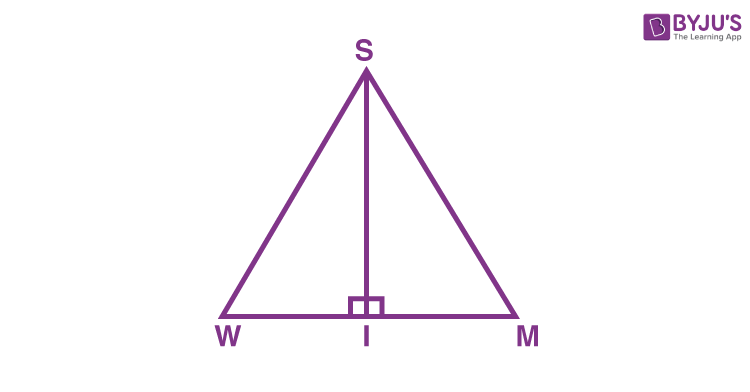In the above figure, the line segment SI is the perpendicular bisector of WM.

## External Angle Bisector Theorem

The external angle bisector of a triangle divides the opposite side externally in the ratio of the sides containing the angle. This condition occurs usually in non-equilateral triangles.

### Proof

Given: In ΔABC, AD is the external bisector of ∠BAC and intersects BC produced at D.

To prove : BD/DC  =  AB/AC

Construction: Draw CE ∥ DA meeting AB at E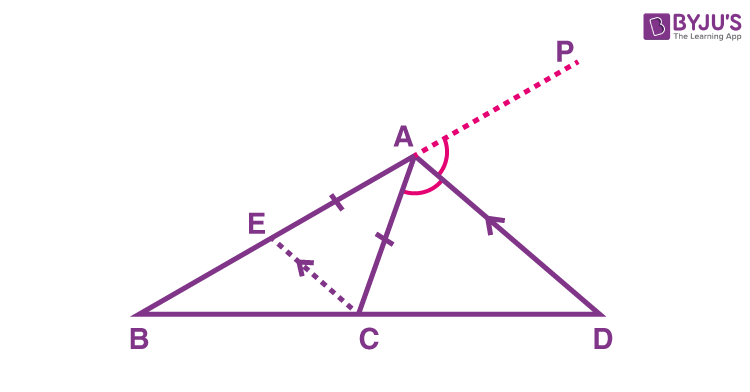Since, CE ∥ DA and AC is transversal, therefore,

∠ECA  =  ∠CAD (alternate angles)   ……(1)

Again, CE ∥ DA and BP is a transversal, therefore,

∠CEA  =  ∠DAP (corresponding angles) —–(2)

But AD is the bisector of ∠CAP,

As we know, Sides opposite to equal angles are equal, therefore,

∠CEA  =  ∠ECA

BD/DC  =  BA/AE   [By Thales Theorem]

AE  =  AC,

BD/DC  =  BA/AC

Hence, proved.

## Solved Examples on Angle Bisector Theorem

Go through the following examples to understand the concept of the angle bisector theorem.

Example 1:

Find the value of x for the given triangle using the angle bisector theorem.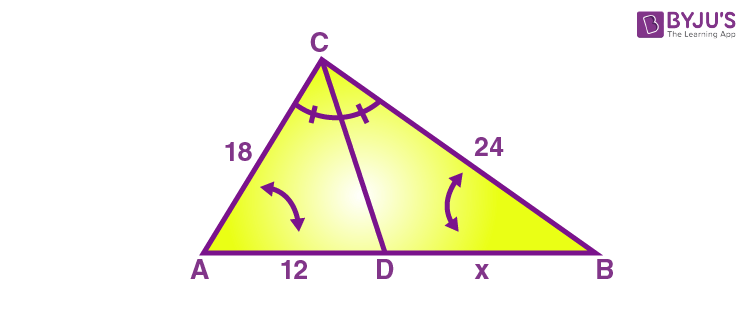Solution:

Given that,

AD = 12, AC = 18, BC=24, DB = x

According to angle bisector theorem,

Now substitute the values, we get

12/18 = x/24

X = (⅔)24

x = 2(8)

x= 16

Hence, the value of x is 16.

Example 2:

ABCD is a quadrilateral in which the bisectors of angle B and angle D intersects on AC at point E. Show that AB/BC = AD/DC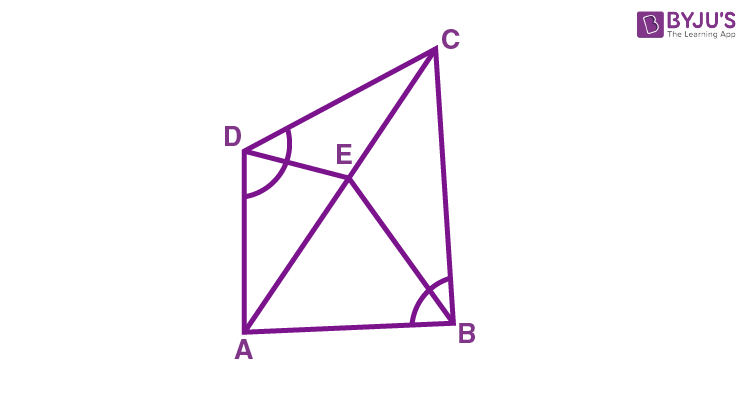Solution:

From the given figure, the segment DE is the angle bisector of angle D and BE is the internal angle bisector of angle B.

Hence, the using internal angle bisector theorem, we get

Similarly,

AE/EC = AB/BC ….(2)

From equations (1) and (2), we get

Hence, AB/BC = AD/DC is proved.

Example 3.

In a triangle, AE is the bisector of the exterior ∠CAD that meets BC at E. If the value of AB = 10 cm, AC = 6 cm and BC = 12 cm, find the value of CE.

Solution:

Given : AB = 10 cm, AC = 6 cm and BC = 12 cm

Let CE is equal to x.

By exterior angle bisector theorem, we know that,

BE / CE = AB / AC

(12 + x) / x = 10 / 6

6( 12 + x ) = 10 x [ by cross multiplication]

72 + 6x = 10x

72 = 10x – 6x

72 = 4x

x = 72/4

x = 18

CE = 18 cm

To learn more important Maths theorems, visit BYJU’S – The Learning App and learn all the concepts easily.

## Frequently Asked Questions on Angle Bisector Theorem

Q1

### What does the angle bisector theorem state?

According to the angle bisector theorem, an angle bisector of an angle of a triangle divides the opposite side into two parts that are proportional to the other two sides of the triangle.
Q2

### What is the formula of angle bisector?

In the triangle ABC, the angle bisector intersects side BC at point D. Thus,
BD/DC = AB/AC
Q3

### The angle bisector of vertex angle of an isosceles triangle bisects the opposite side. True or False.

True. An isosceles triangle has two pairs of equal sides with a common vertex. If the angle bisector of vertex angle is drawn, then it divides the opposite side into equal parts.
Q4

### How to find the angle bisector of an angle?

Draw an angle say ∠ABC, angled at B. Using a compass, and taking B as centre and any radius, draw an arc intersecting BA at P and BC at Q, respectively. Now taking P and Q as the centre and with the same radius, draw two arcs intersecting each other at R. Join the vertex B to point R and draw the ray BR. Thus, BR is the angle bisector of ∠ABC.